Click to Chat

1800-1023-196

+91-120-4616500

CART 0

• 0

MY CART (5)

Use Coupon: CART20 and get 20% off on all online Study Material

ITEM
DETAILS
MRP
DISCOUNT
FINAL PRICE
Total Price: Rs.

There are no items in this cart.
Continue Shopping• Complete JEE Main/Advanced Course and Test Series
• OFFERED PRICE: Rs. 15,900
• View Details

Revision Notes on Area under Curves

Asymptotes are the lines whose distance from the curve tends to zero as the point on the curve moves towards infinity along the branch of the curve.

Conditions for various asymptotes:

1) If lim x→a f(x) = ∞ or lim x→a f(x) = -∞, then x = a is an asymptote of y = f(x).

2) If lim x→ +∞ f(x) = k or lim x→ -∞ f(x) = k, then y = k is an asymptote of y = f(x).

3) If lim x→-∞ f(x)/x = a2 and lim x→-∞ (f(x) – a2x) = c*, then y = a2x + c* is an asymptote which is inclined towards the left.

4) If lim x→∞ f(x)/x = a1 and lim x→∞ (f(x) – a1x) = c, then y = a1x + c is an asymptote which is inclined towards the right.

In order to compute the area of different curves, we first need to find the approximate shape of the curves which can be determined as:

1) If all the powers of y in the equation of curve are even, then the curve is symmetrical about the x-axis. For eg: y2 = 4ax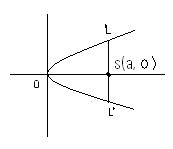2) Similarly, if all the powers of y in the equation of curve are even, then the curve is symmetrical about the y-axis. For eg: x2 = 4ay3) If all the powers of x and y in the equation of the curve are even, then the curve is symmetrical both about x as well as y-axis. Eg: x2 + y2 = a24) If the equation of the curve remains unchanged on interchanging x and y, then the curve is symmetrical about the line y = x. Eg: x3 + y3 = 3axy5) If the equation of the curve remains unaltered when x and y are replaced by their negatives i.e. –x and –y respectively, the curve is symmetric in opposite quadrants. Eg: xy = c2

Area bounded by the curves y = f(x) above x-axis and between the lines x = a and x = b: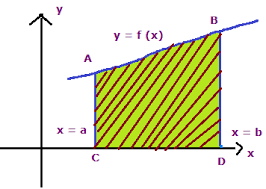In the above case, if the area between the curves x = a and x = b lies below the x-axis, then Area bounded by the curves y = f(x) and the lines x = a and x = b: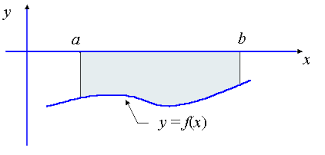The area of the curve lying between y = c and y = d on the left of y-axis is given by

If F(x) ≥ G(x) in [a, c] and F(x) ≤ G(x) in [c, b] where a < c < b, then area of the region bounded by the curve is given by

Calculation of area by integration between the curves y = Φ(x) and y = Ψ(x) and ordinates x = x1 and x = x2:

1) To determine the area between curves, first find out the points of intersection of the two curves.2) If in the domain common to both (i.e. the domain given by the points of intersection) the curves lie above x-axis, then area is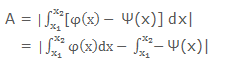3) If some part of both the curves lies below x-axis then the individual integral must be calculated according to the case in question.

Area between curve y = f(x) and y-axis:

1)In order to obtain area between y = f(x) and the y-axis, the function should first be written in y i.e. y = f(x) must be converted to x = g(y).

2) The integral should then be evaluated as ∫xdy or ∫ydx where integral runs from y1 to y23) The area bounded between the y-axis and the curves y = f(x) and y = g(x) can be determined on similar lines by first locating the enclosed region which also  helps in identifying the limits.

Some chief points to be kept in mind:

1) In case, the curve is completely above the x-axis, the resulting area is positive but on the other hand, if it lies completely below the x-axis the area would be negative

2) By, convention only the magnitude is taken into consideration.

3) At times, the curve lies partly above and partly below the x-axis. So in such cases both the areas should be computed separately. Then add up their moduli to get the resultant area.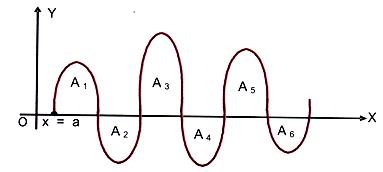4) If the curve y = f(x) crosses the x-axis n times when x varies from a to b, then the areas between y = f(x), the x-axis and the lines x= a and x = b is given by A = |A1| + |A2| +……+ |An|.

5) If the curve is symmetrical about the x-axis, or the y-axis, or both, then calculate the area of one symmetrical part and multiply it by the number of symmetrical parts to get the whole area.

Some other basic cases:

1) Let f(x) be a continuous function in (a, b). Then the area bounded by the curve y = f(x), the x-axis and the lines x = a and x = b is given by the formulae A = | ∫ab f(x) dx |, provided f(x) > 0 (or f(x) < 0) ∀ x ∈ (a, b).

2) The area bounded by x = f(y), the y-axis and the lines y = c and y = d is given by A = | ∫cd f(y) dy |, provided f(y) > 0 or f(y) < 0 ∀ y ∈ (c, d)

3) If we have two functions f(x) and g(x) such that f(x) < g(x) ∀ x ∈ [a, b], then the area bounded by the curves y = f(x), y = g(x) and the lines x = a, x = b (a < b) is given by A = | ∫ab [g(x) – f(x)] dx|.

4) If point (0, 0) satisfies the equation, the curve passes through origin.

5) If curve passes through origin, then equate the lowest degree term to zero and get the equation of tangent. In case there are two tangents, the origin is a double point.

6) The points of inflexion can be identified by equation d2y/dx2 = 0 or d2x/dy2 = 0 and solving the resulting equation.### Course Features

• 728 Video Lectures
• Revision Notes
• Previous Year Papers
• Mind Map
• Study Planner
• NCERT Solutions
• Discussion Forum
• Test paper with Video Solution